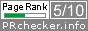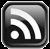# LEARN MS-ACCESS TIPS AND TRICKS

Learn Microsoft Access Advanced Programming Techniques, Tips and Tricks.

### Introduction

This is all about exporting data into Text File and sending it, through the Internet as an E-mail attachment, to a remote location.  At the receiving end, the Text data is converted back into its original form and added to an existing table.  No field delimiters, field names, or any other details are sent along with the actual data lines.  The Source and Target Tables should have identical Structure.

Let us take a quick look at some sample data and how they look like when converted into Text Format.

#### Sample MS-Access Table: Export

MS-Access Table: Export text data image, when exported into Text File: Export.txt.

As you can see from the above text image that each record from Access Table: Export is converted into a continuous stream of characters and written into the Text File in separate lines.  The Text File is saved with the same name as the Table: Export with the file extension .txt (Export.txt).

The first two data fields on the Source Table are Text Fields (size 15 characters each), the third field is Date type and the next five fields are of Numeric Type.

Data Field details are given below:

Seq Field Type Size
1. LastName Text 15
2. FirstName Text 15
3. DofB Date
4. Height Number Integer
5. Weight Number Long Integer
6. H Number Single
7. W Number Double
8. Dcml Number Decimal

#### The VBA txtExport() Function Code

The VBA program given below reads the MS-Access Table, record by record, converts them into text format, and writes out into an external Text File, with the same name as the Source Table.  The text file:Export.txt is created in the Default Database Folder.  I have created the sample data Table with the name Export for Demo purposes only.  You can use any Table from your database, but see that it doesn’t contain any field types other than text, date, or Number.

```Public Function txtExport(ByVal tblName As String)
'-----------------------------------------------------
'Purpose: Export Data into Text Format
'Author : a.p.r.pillai
'Date   : June, 2013
'Remarks: All Rights Reserved by www.msaccesstips.com
'-----------------------------------------------------
'Data Types
'----------
'Text - 10 = Actual size
'Date - 8 = 10 characters
'Integer - 3
'Long Integer - 4
'Single - 6
'Double - 7
'Decimal - 20
'Numeric types 3,4,6,7 or 20 = 12 characters

Dim tblSize() As Variant
Dim db As Database, rst As Recordset
Dim fld As Field, fldCount As Integer
Dim j As Integer, tbldef As TableDef
Dim numSize As Integer, dtSize As Integer
Dim fmt As String, outTxt As String
Dim outFileName As String

On Error GoTo txtExport_Err

numSize = 12
dtSize = 10

'Exported Text Filename is same as Tablename with file-extension .Txt
outFileName = tblName & ".txt"

Set db = CurrentDb
Set tbldef = db.TableDefs(tblName)
fldCount = tbldef.Fields.Count - 1
'A singly dimensioned Array of Variant Type
'is decalred for number of fields in the Table.
ReDim tblSize(fldCount)

'The Array is initialized with text data type
'for appropriate size to hold text, date & Numeric Values
For j = 0 To fldCount
Set fld = tbldef.Fields(j)
Select Case fld.Type
Case 3, 4, 6, 7, 20 'Numeric data type
tblSize(j) = String(numSize, "0")
Case 8 'Date Data type
tblSize(j) = String(dtSize, "0")
Case 10 'Text data type
tblSize(j) = String(fld.Size, "x") 'Actual Text Field-size
End Select Next

'Open the Source Table
Set rst = db.OpenRecordset(tblName)
'Create and open the output text file in the same folder of the database
Open outFileName For Output As #1
'Read records till the end of Table and convert them into text format
Do While Not rst.EOF
For j = 0 To fldCount
Set fld = tbldef.Fields(j)
Select Case fld.Type
Case 3, 4, 6, 7, 20
fmt = "00000000.000"
'position data right-aligned into the text variable
RSet tblSize(j) = Format(rst.Fields(j).Value, fmt)
Case 8
fmt = "dd/mm/yyyy"
'position data right-aligned into the text variable
RSet tblSize(j) = Format(rst.Fields(j).Value, fmt)
Case 10
'position data left-aligned into the text variable
LSet tblSize(j) = rst.Fields(j).Value
End Select
Next
outTxt = ""
For j = 0 To fldCount
'join all text data variables into a single text line
outTxt = outTxt & tblSize(j)
Next
'Write into text file
Print #1, outTxt
'take next record to export
rst.MoveNext
Loop

'Close the Text File and other files
Close #1
rst.Close
db.Close

Set db = Nothing
Set tbldef = Nothing
Set fld = Nothing

txtExport_Exit:
Exit Function

txtExport_Err:
MsgBox Err & " : " & Err.Description, , "txtExport()"
Resume txtExport_Exit
End Function```

#### Running the Code

You can Run the Program directly from the Debug Window Command line:

Syntax: txtExport "Table Name"

`txtExport "Export"`

Or run it from a Command Button Click Event Procedure

`Private Sub cmdRun_Click()txtExport "Export"End Sub`

### The Data Format Change.

1. When Text Type Field values are converted into output text the actual size of the field is calculated and the data is left-aligned within the actual size of the text field. If the field value is shorter than the actual size of the field then balance character positions are filled with spaces.
2. Date Field value uses 10 characters (dd/mm/yyyy) when converted into text.
3. All Numeric Field Values are converted into a 12 character text type and positioned Right-aligned in the output memory image, filled with zeroes at left positions.

NB: Date and Numeric Data text field sizes (Date=10, Number=12) are selected arbitrarily and can be modified if needed.  The MS-Access Table should have only the above three types of Data (Text, Date & Number) Fields in it.

The Text File created from MS-Access Table can be sent through E-Mail Attachment to the remote location.  The VBA Program given below can be used for converting the Text File back into Access Data and append into the Table with the same structure as the Source Table.

```Public Function txtImport(ByVal txtFileName As String)
'txtFileName is same as Tablename with file-extension .txt
'-----------------------------------------------------
'Purpose: Import Text-Data into Table
'Author : a.p.r.pillai
'Date   : June, 2013
'Remarks: All Rights Reserved by www.msaccesstips.com
'-----------------------------------------------------
'Data Types
'----------
'Text - 10 = Actual size
'Date - 8 = 10 characters
'Integer - 3
'Long Integer - 4
'Single - 6
'Double - 7
'Decimal - 20
'Numeric types 3,4,6,7 or 20 = 12 characters

Dim tblSize() As Variant
Dim db As Database, rst As Recordset
Dim fld As Field, fldCount As Integer
Dim j As Integer, tbldef As TableDef
Dim numSize As Integer, dtSize As Integer
Dim fmt As String, outTxt As String
Dim inputFileName As String, I As Integer, k As Integer

On Error GoTo txtImport_Err

numSize = 12
dtSize = 10

inputFileName = txtFileName & ".txt"
Set db = CurrentDb
Set tbldef = db.TableDefs(txtFileName)
fldCount = tbldef.Fields.Count - 1

'A singly dimensioned Array of Variant Type
'is decalred for number of fields in the Table.
ReDim tblSize(fldCount)

'The Array is initialized with text data type
'for appropriate size to hold text, date & Numeric Values
For j = 0 To fldCount
Set fld = tbldef.Fields(j)
Select Case fld.Type
Case 3, 4, 6, 7, 20 'Numeric data type
tblSize(j) = String(numSize, "0")
Case 8 'Date data type
tblSize(j) = String(dtSize, "0")
Case 10 'Text Field
tblSize(j) = String(fld.Size, "x") ' Actual Text Field-size
End Select
Next

'Open the Target Table Export
Set rst = db.OpenRecordset(txtFileName)

'Open the input text file
Open inputFileName For Input As #1

'Read the Text file, convert and add the data to the Target Table
Do While Not EOF(1)
'Read text data line
Input #1, outTxt
I = 1 'first field value off-set
'Add a new record into the table
rst.AddNew

For j = 0 To fldCount
Set fld = tbldef.Fields(j)
'read data size for extracting correct number of characters
'from the text line
k = Len(tblSize(j))

Select Case fld.Type 'check the current field type
Case 3, 4, 6, 7, 20 'if numeric data type then
'Extract numeric data, convert and write into data field
rst.Fields(j).Value = Val(Mid(outTxt, I, k))
I = I + k 'increment to next field off-set
Case 8 'if it is date then
'Extract Date data, convert and write into data field
rst.Fields(j).Value = CDate(Mid(outTxt, I, k))
I = I + k 'increment to next field off-set
Case 10 'Text data type
'Extract Date data, convert and write into data field
rst.Fields(j).Value = Mid(outTxt, I, k)
I = I + k 'increment to next field off-set
End Select
Next
rst.Update
'initialize the variable to read the next line of text data
outTxt = ""
Loop

'No more text data in the file
Close #1
rst.Close
db.Close
Set db = Nothing
Set tbldef = Nothing
Set fld = Nothing

txtImport_Exit:
Exit Function

txtImport_Err:
MsgBox Err & " : " & Err.Description, , "txtImport()"
Resume txtImport_Exit

End Function```

Debug Window Commandline Run Syntax:

txtImport "Text File Name"

`txtImport "Export"`

NB: Text File Name is entered without the file extension (.TXT). Target Table should have the same structure as the Source Table.

Share:

#### Post a Comment

Comments subject to moderation before publishing.

## TranslateSubscribe in a readerYour email address:

Delivered by FeedBurner